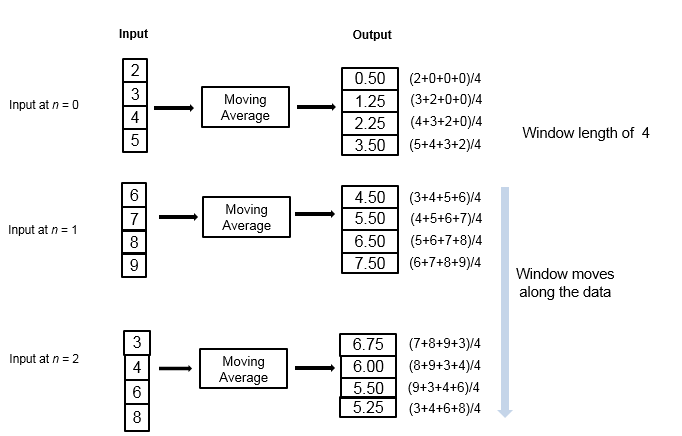## What Are Moving Statistics?

You can measure statistics of streaming signals in MATLAB® and Simulink® along each independent data channel using the moving statistics System objects and blocks. Statistics such as average, RMS, standard deviation, variance, median, maximum, and minimum change as the data changes constantly with time. With every data sample that comes in, the System objects and blocks compute the statistics over the current sample and a specific window of past samples. This window "moves" as new data comes in.

These System objects and blocks compute the moving statistic using one or both of the sliding window method and exponential weighting method. For more details on these methods, see Sliding Window Method and Exponential Weighting Method.

Consider an example of computing the moving average of a streaming input data using the sliding window method. The algorithm uses a window length of 4. At the first time step, the algorithm fills the window with three zeros to represent the first three samples. In the subsequent time steps, to fill the window, the algorithm uses samples from the previous data frame. The moving statistic algorithms have a state and remember the previous data.If the data is stationary, use the stationary statistics blocks to compute the statistics over the entire data in Simulink. Stationary blocks include Autocorrelation, Correlation, Maximum, Mean, Median, Minimum, RMS, Sort, Standard Deviation, and Variance.

These blocks do not maintain a state. When a new data sample comes in, the algorithm computes the statistic over the entire data and has no influence from the previous state of the block.

Consider an example of computing the stationary average of streaming input data using the Mean block in Simulink. The Mean block is configured to find the mean value over each column.At each time step, the algorithm computes the average over the entire data that is available in the current time step and does not use data from the previous time step. The stationary statistics blocks are more suitable for data that is already available rather than for streaming data.

Watch now